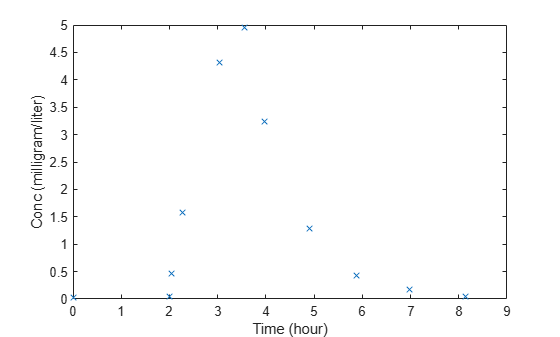Main Content

# LagParameterName

Parameter specifying time lag for dose

## Description

`LagParameterName` is a property of a `RepeatDose` or `ScheduleDose` object.

Specify the name of a parameter object that is scoped to a model. The parameter defines the length of time it takes for the dose to reach its target after being introduced.

You can parameterize the property by setting it to the name of a model-scoped parameter that is not being modified by a repeated assignment rule, an algebraic rule, or a rate rule. However, the parameter can be modified by an event.

## Characteristics

 Applies to Objects: `RepeatDose`, `ScheduleDose`. Data type Character vector. Data values Name of a model-scoped parameter object. The default value is an empty character vector `''`. Access Read/write.

## Examples

expand all

This example shows how to estimate the time lag before a bolus dose was administered and the duration of the dose using a one-compartment model.

Load a sample data set.

`load lagDurationData.mat`

Plot the data.

```plot(data.Time,data.Conc,'x') xlabel('Time (hour)') ylabel('Conc (milligram/liter)')```Convert to groupedData.

```gData = groupedData(data); gData.Properties.VariableUnits = {'hour','milligram/liter'};```

Create a one-compartment model.

```pkmd = PKModelDesign; pkc1 = addCompartment(pkmd,'Central'); pkc1.DosingType = 'Bolus'; pkc1.EliminationType = 'linear-clearance'; pkc1.HasResponseVariable = true; model = construct(pkmd); configset = getconfigset(model); configset.CompileOptions.UnitConversion = true;```

Add two parameters that represent the time lag and duration of a dose. The lag parameter specifies the time lag before the dose is administered. The duration parameter specifies the length of time it takes to administer a dose.

```lagP = addparameter(model,'lagP'); lagP.ValueUnits = 'hour'; durP = addparameter(model,'durP'); durP.ValueUnits = 'hour';```

Create a dose object. Set the `LagParameterName` and `DurationParameterName` properties of the dose to the names of the lag and duration parameters, respectively. Set the dose amount to 10 milligram which was the amount used to generate the data.

```dose = sbiodose('dose'); dose.TargetName = 'Drug_Central'; dose.StartTime = 0; dose.Amount = 10; dose.AmountUnits = 'milligram'; dose.TimeUnits = 'hour'; dose.LagParameterName = 'lagP'; dose.DurationParameterName = 'durP';```

Map the model species to the corresponding data.

`responseMap = {'Drug_Central = Conc'};`

Specify the lag and duration parameters as parameters to estimate. Log-transform the parameters. Initialize them to 2 and set the upper bound and lower bound.

```paramsToEstimate = {'log(lagP)','log(durP)'}; estimatedParams = estimatedInfo(paramsToEstimate,'InitialValue',2,'Bounds',[1 5]);```

Perform parameter estimation.

`fitResults = sbiofit(model,gData,responseMap,estimatedParams,dose,'fminsearch')`
```fitResults = OptimResults with properties: ExitFlag: 1 Output: [1x1 struct] GroupName: One group Beta: [2x4 table] ParameterEstimates: [2x4 table] J: [11x2 double] COVB: [2x2 double] CovarianceMatrix: [2x2 double] R: [11x1 double] MSE: 0.0024 SSE: 0.0213 Weights: [] LogLikelihood: 18.7511 AIC: -33.5023 BIC: -32.7065 DFE: 9 DependentFiles: {1x2 cell} EstimatedParameterNames: {'lagP' 'durP'} ErrorModelInfo: [1x3 table] EstimationFunction: 'fminsearch' ```

Display the result.

`fitResults.ParameterEstimates`
```ans=2×4 table Name Estimate StandardError Bounds ________ ________ _____________ ______ {'lagP'} 1.986 0.0051568 1 5 {'durP'} 1.527 0.012956 1 5 ```
`plot(fitResults)`## SupportGet trial now